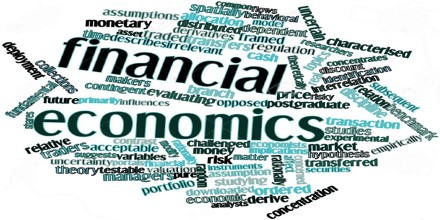Theory of Financial Economics

Introduction

Financial Economics is a branch of economics that analyzes the use and distribution of resources in markets in which decisions are made under uncertainty. Its concern is thus the interrelation of financial variables, such as prices, interest rates and shares, as opposed to those concerning the real economy. It has two main areas of focus: asset pricing (or “investment theory”) and corporate finance; the first being the perspective of providers of capital and the second of users of capital. Financial economics employs economic theory to evaluate how time, risk (uncertainty), opportunity costs and information can create incentives or disincentives for a particular decision.

Financial economics often involves the creation of sophisticated models to test the variables affecting a particular decision. Often, these models assume that individuals or institutions making decisions act rationally, though this is not necessarily the case. Irrational behavior of parties has to be taken into account in financial economics as a potential risk factor.Financial econometrics is the branch of financial economics that uses econometric techniques to parameterise these relationships. Mathematical finance is related in that it will derive and extend the mathematical or numerical models suggested by financial economics. Note though that the emphasis there is mathematical consistency, as opposed to compatibility with economic theory.

The paradigms of financial economics, which is sometimes also called modern finance, are the result of a type of academic thinking inspired by a positivist philosophy.  This philosophy has been based on Milton Friedman’s (1953) now-famous essay, “The Methodology of Positive Economics,” and later modified by other well known researchers, including Robert Lucas and Eugene Fama, to fit their narrowly defined purpose.  The most significant financial economics paradigms are also imbued with the basic canons of neoclassical economics and the belief that the firm exists for no reason other than the economic pleasure of the shareholder.  These paradigms include the efficient markets hypotheses, the capital asset pricing model, and the Modigliani and Miller irrelevance theorems (Modigliani and Miller, 1958; and Miller and Modigliani, 1961).Present Value, Expectation and Utility

Underlying all of financial economics are the concepts of present value and expectation. Calculating their present value allows the decision maker to aggregate the cashflows or other returns to be produced by the asset in the future, to a single value at the date in question, and to thus more readily compare two opportunities; this concept is therefore the starting point for financial decision making. This decision method, however, fails to consider risk aversion. In other words, since individuals receive greater utility from an extra dollar when they are poor and less utility when comparatively rich, the approach is to therefore “adjust” the weight assigned to the various outcomes (“states”) correspondingly. Some investors may in fact be risk seeking as opposed to risk averse, but the same logic would apply.

Arbitrage-Free Pricing

The concepts of arbitrage-free, “rational”, pricing and equilibrium are then coupled with the above to derive “classical” financial economics. Rational pricing is the assumption that asset prices and hence asset pricing models will reflect the arbitrage-free price of the asset, as any deviation from this price will be “arbitraged away”. This assumption is useful in pricing fixed income securities, particularly bonds, and is fundamental to the pricing of derivative instruments.State Prices

With the above relationship established, the further specialized Arrow–Debreu model may be derived. This important result suggests that, under certain economic conditions, there must be a set of prices such that aggregate supplies will equal aggregate demands for every commodity in the economy. The analysis here is often undertaken assuming a Representative agent. The concept of a state price security also called an Arrow-Debreu security, a contract that agrees to pay one unit of a numeraire a currency or a commodity if a particular state occurs at a particular time in the future and pays zero numeraire in all the other states. The price of this security is the state price of this particular state of the world.

State prices find immediate application as a conceptual tool; but they can also be applied to valuation problems. Given the pricing mechanism described, one can decompose the derivative value as a linear combination of its state-prices, i.e. back-solve for the state-prices corresponding to observed derivative prices, and these recovered state-prices can then be used for valuation of other instruments with exposure to the underlyer, or for other decision making relating to the underlyer itself.Challenges and Criticism

The first set of challenges: As discussed, the assumptions that market prices follow a random walk and / or that asset returns are normally distributed are fundamental. Empirical evidence, however, suggests that these assumptions may not hold and that in practice, traders, analysts and risk managers frequently modify the “standard models”. In fact, Benoît Mandelbrot had discovered already in the 1960s that changes in financial prices do not follow a Gaussian distribution, the basis for much option pricing theory, although this observation was slow to find its way into mainstream financial economics.

The second set of challenges: As seen, a common assumption is that financial decision makers act rationally; Homo economicus. Recently, however, researchers in experimental economics and experimental finance have challenged this assumption empirically. These assumptions are also challenged theoretically, by behavioral finance, a discipline primarily concerned with the limits to rationality of economic agents.